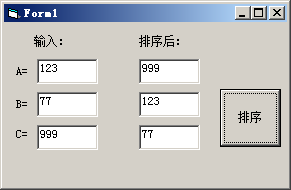﻿ 设A、B、C是同一类型的变量，并分别被赋予不同大小的数据，设计一个算法，使得执行的结果为A>B>C 第二章 答案 新编Visual Basic程序设计教程 陈斌 著 - 济亨网

设A、B、C是同一类型的变量，并分别被赋予不同大小的数据，设计一个算法，使得执行的结果为A>B>C 第二章 答案 新编Visual Basic程序设计教程 陈斌 著

2017-05-29 21:20:45  分类: VB程序题  参与: AddViewCount(146)人

Private Sub Command1_Click()

Dim a As Integer, b As Integer, c As Integer
Dim z As Integer 'z值用于交换用

a = Text1.Text: b = Text2.Text: c = Text3.Text

If a < b Then
z = a: a = b: b = z
End If '只要a<b就交换，保证交换后，a值>b值

If a < c Then
z = a: a = c: c = z
End If '只要a<c就交换，保证交换后，a值>c值；经过两次交换，a已经是三个最大的那个了。

If b < c Then
z = b: b = c: c = z
End If '只要b<c就交换，保证交换后，b值>c值；即b值是第二大的值

Text4.Text = a
Text5.Text = b
Text6.Text = c

End Sub---- 新编Visual Basic程序设计教程   答案 陈斌  著

<< 上一篇下一篇 >>